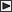■建築研究報告

 Room Temperature in Fire of a Fire-Resistive Room Takasi SEKINE 建築研究報告  No.29,  1959  建設省建築研究所

 ＜概要＞ In the case where a fire-resistive room is filled completely with flame, the temperature gradient in the room can be estimated according to its window area and wall area. The purpose of this paper is to obtain room temperature from the equation of heat balance. Since the heat quantity transmitted from flame to wall surface by thermal radiation is generally so greater than that of convection, heat quantity by radiation is discussed separately in Section II. The equation of heat balance of fire in room is explained in Section III. Solving the equation of heat balance in successive way, the temperature gradient in the rooms with various window area are obtained. When the ratio of window area to wall area (AB/AT) and the window height (W) are given, the temperature gradient is obtained as shown in Fig. 4. It is obvious that the temperature gradient varies depending upon the height and area of window. As Fig. 5 shows the room temperature at the most active combustion in fire (tGM) is obtained, if (W) and (AB/AT) are given, where tGM is defined as the temperature at 30 min. after the ignition.   Temperature tGM, as shown in Table 2, agrees with the measured one obtained in the full-scale experimental fires.所在地・交通案内関連リンクサイトマップお問い合わせリンク・著作権